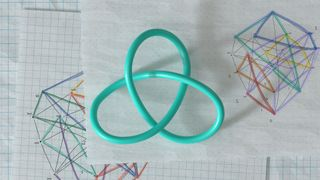# DeepMind cracks 'knot' conjecture that bedeviled mathematicians for decadesThe artificial intelligence (AI) program DeepMind has gotten closer to proving a math conjecture that's bedeviled mathematicians for decades and revealed another new conjecture that may unravel how mathematicians understand knots.

The two pure math conjectures are the first-ever important advances in pure mathematics (or math not directly linked to any non-math application) generated by artificial intelligence, the researchers reported Dec. 1 in the journal Nature. Conjectures are mathematical ideas that are suspected to be true but have yet to be proven in all circumstances. Machine-learning algorithms have previously been used to generate such theoretical ideas in mathematics, but thus far these algorithms have tackled problems smaller than the ones DeepMind has cracked.

"What hasn't happened before is using [machine learning] to make significant new discoveries in pure mathematics," said Alex Davies, a machine-learning specialist at DeepMind and one of the authors of the new paper.

## Math and machine learning

Much of pure mathematics is noticing patterns in numbers and then doing painstaking numerical work to prove whether those intuitive hunches represent real relationships. This can get quite complicated when working with elaborate equations in multiple dimensions.

However, "the kind of thing that machine learning is very good at, is spotting patterns," Davies told Live Science.

The first challenge was setting DeepMind onto a useful path. Davies and his colleagues at DeepMind worked with mathematicians Geordie Williamson of the University of Sydney, Marc Lackenby of the University of Oxford, and András Juhász, also of the University of Oxford, to determine what problems AI might be useful for solving.

They focused on two fields: knot theory, which is the mathematical study of knots; and representation theory, which is a field that focuses on abstract algebraic structures, such as rings and lattices, and relates those abstract structures to linear algebraic equations, or the familiar equations with Xs, Ys, pluses and minuses that might be found in a high-school math class.

## Knotty problems

In understanding knots, mathematicians rely on something called invariants, which are algebraic, geometric or numerical quantities that are the same. In this case, they looked at invariants that were the same in equivalent knots; equivalence can be defined in several ways, but knots can be considered equivalent if you can distort one into another without breaking the knot. Geometric invariants are essentially measurements of a knot's overall shape, whereas algebraic invariants describe how the knots twist in and around each other.

"Up until now, there was no proven connection between those two things," Davies said, referring to geometric and algebraic invariants. But mathematicians thought there might be some kind of relationship between the two, so the researchers decided to use DeepMind to find it.

With the help of the AI program, they were able to identify a new geometric measurement, which they dubbed the "natural slope" of a knot. This measurement was mathematically related to a known algebraic invariant called the signature, which describes certain surfaces on knots.

The new conjecture — that these two types of invariants are related — will open up new theorizing in the mathematics of knots, the researchers wrote in Nature.

In the second case, DeepMind took a conjecture generated by mathematicians in the late 1970s and helped reveal why that conjecture works.

For 40 years, mathematicians have conjectured that it's possible to look at a specific kind of very complex, multidimensional graph and figure out a particular kind of equation to represent it. But they haven't quite worked out how to do it. Now, DeepMind has come closer by linking specific features of the graphs to predictions about these equations, which are called Kazhdan–Lusztig (KL) polynomials, named after the mathematicians who first proposed them.

"What we were able to do is train some machine-learning models that were able to predict what the polynomial was, very accurately, from the graph," Davies said. The team also analyzed what features of the graph DeepMind was using to make those predictions, which got them closer to a general rule about how the two map to each other. This means DeepMind has made significant progress on solving this conjecture, known as the combinatorial invariance conjecture.

There are no immediate practical applications for these pure math conjectures, but the mathematicians plan to build on the new discoveries to uncover more relationships in these fields. The research team is also hopeful that their successes will encourage other mathematicians to turn to artificial intelligence as a new tool.

"The first thing we'd like to do is go out there into the mathematical community a little bit more and hopefully encourage people to use this technique and go out there and find new and exciting things," Davies said.

Originally published on Live Science